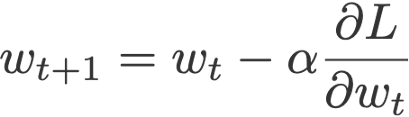Join thousands of students in our LangChain and Vector DBs in Production course, with over 50+ lessons and practical projects for FREE!.

## PublicationDeep Learning

# Improve Your ML Models Training

Last Updated on January 6, 2023 by Editorial Team

#### Cycling learning rates in Tensorflow 2.0

Deep learning has found its way into all kinds of research areas in the present times and has also become an integral part of our lives. The words of Andrew Ng help us to sum it up really well,

Artificial Intelligence is the new electricity.”

However, with any great technical breakthroughs come a large number of challenges too. From Alexa to Google Photos to your Netflix recommendations, everything at its core is just deep learning, but it comes with a few hurdles of its own:

• Availability of huge amounts of data
• Availability of suitable hardware for high performance
• Overfitting on available data
• Lack of transparency
• Optimization of hyperparameters

### The problem with the typical approach:

A deep neural network usually learns by using stochastic gradient descent and the parameters θ (or weights ω) are updated as follows:

where L is a loss function and α is the learning rate.

We know that if we set the learning rate too small, the algorithm will take too much time to converge fully, and if it’s too large, the algorithm will diverge instead of converging. Hence, it is important to experiment with a variety of learning rates and schedules to see what works best for our model.

In practice, there are a few more problems which arise due to this method:

• The deep learning model and optimizer are sensitive to our initial learning rate. A bad choice of the starting learning rate can greatly hamper the performance of our model from the beginning itself.
• It could lead to a model that is stuck at local minima or in a saddle point. When that happens, we may not be able to descend to a place of lower loss even if we keep on lowering our learning rate further.

### Cyclic Learning Rates help us overcome these problems

Using Cyclical Learning Rates you can dramatically reduce the number of experiments required to tune and find an optimal learning rate for your model.

Now, instead of monotonically decreasing the learning rate, we:

1. Define a lower bound on our learning rate (base_lr).
2. Define an upper bound on the learning rate (max_lr).

So the learning rate oscillates between these two bounds while training. It slowly increases and decreases after every batch update.

With this CLR method, we no longer have to manually tune the learning rates and we can still achieve near-optimal classification accuracy. Furthermore, unlike adaptive learning rates, the CLR method requires no extra computation.

The improvement will be clear to you by seeing an example.

### Implementing CLR on a dataset

Now we will train a simple neural network model and compare the different optimization techniques. I have used here a dataset on Cardiovascular disease.

These are all the imports you’ll need over the course of the implementation:

And this is what the data looks like:

The column cardio is the target variable, and we perform some simple scaling of the data and split it into features (X_data) and targets (y_data).

Now we use train_test_split to get a standard train to test ratio of 80–20. Then we define a very basic neural network using aSequential model from Keras. I have used 3 dense layers in my model, but you can experiment with any number of layers or activation functions of your choice.

### Training without CLR:

Here I have compiled the model using the basic ‘SGD’ optimizer which has a default learning rate of 0.01. The model is then trained over 50 epochs.

To show you just the last few epochs, the model takes 3s per epoch and in the end, gives 64.1% training accuracy and 64.7% validation accuracy. In short, this is the result our model gives us after ~150 seconds of training:

### Training using CLR:

Now we use Cyclical Learning Rates and see how our model performs. TensorFlow has this optimizer already built-in and ready to use for us. We call it from the TensorFlow Addons and define it as follows:

The value of step_size can be easily computed from the number of iterations in one epoch. So here, iterations per epoch

= (no. of training examples)/(batch_size)

= 70000/350

= 200.

“experiments show that it often is good to set stepsize equal to 2 − 10 times the number of iterations in an epoch.”¹

Now compiling our model using this newly defined optimizer,

we see that now our model trains much faster, taking even less than 50 seconds in total.

Loss value converges faster and oscillates slightly in the CLR model as we would expect.

Training accuracy has increased from 64.1% to 64.3%.

Testing accuracy also improves, from 64.7% to 65%.

### Conclusion

When you start working with any new dataset, the same values of learning rates you used in previous datasets will not work for your new data. So you have to perform an LR Range Test which gives you a good range for learning rates suitable for your data. Then you can compare your CLR with a fixed learning rate optimizer, as we saw above, to see what suits best to the performance goal you have. So to get this optimal range for the learning rate, you can run the model on a less number of epochs as long as the learning rate keeps increasing linearly. Then oscillating the learning rate between these bounds will be enough to give you a close to optimal result in a few iterations itself.

This optimization technique is clearly a boon as we no longer have to tune the learning rate ourselves. We achieve better accuracy in fewer iterations.

### References:Improve Your ML Models Training was originally published in Towards AI — Multidisciplinary Science Journal on Medium, where people are continuing the conversation by highlighting and responding to this story.

Published via Towards AI